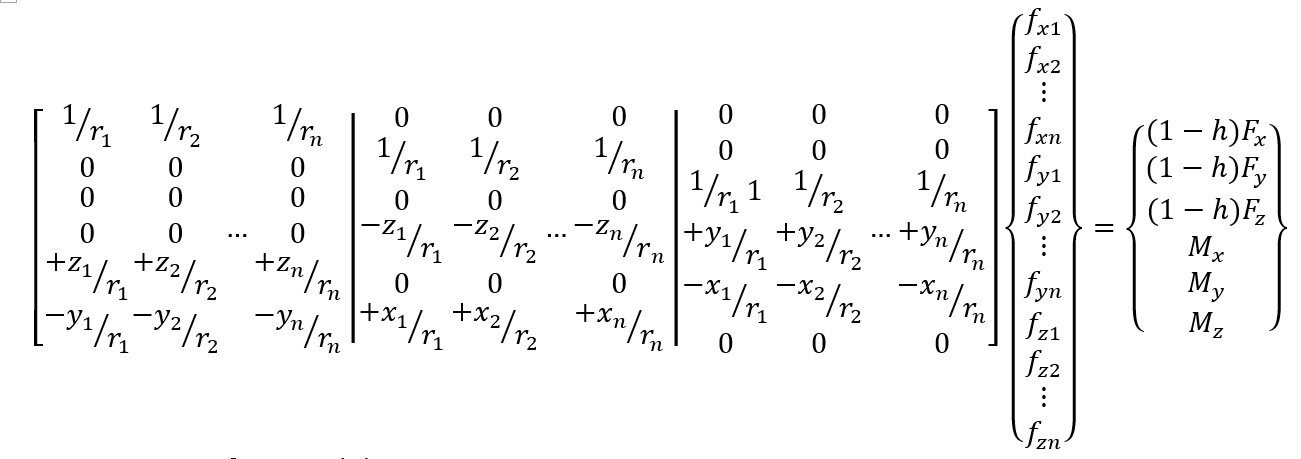Distributed Point Load (DPL)

There are occasions where one wishes to apply ‘point’ moments to a continuum finite element model. This is not directly possible since solid elements do not have rotational degrees of freedom. It is, however, possible by applying appropriate nodal forces to a small cloud of nodes surrounding the node of interest, i.e., the one where the point loads are to be applied, in a statically equivalent manner. DPL automates this task for the practising engineer and the installer for this free software application can be downloaded by clicking on the following image.

DPL needs to solve the following system of equations which is generally underdetermined with a coefficient matrix that may be rank deficient. Conventional matrix decomposition methods fall down with such matrices and so singular value decomposition is used to provide a unique least squares solution to the problem.DOI Number: 10.13140/RG.2.2.24559.07842